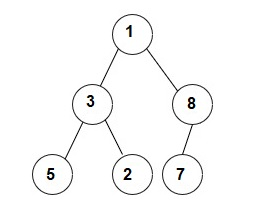New update is available. Click here to update.

# Sibling Nodes

Posted: 26 Feb, 2021
Difficulty: Moderate

## PROBLEM STATEMENT

#### You have been given a Binary Tree of ‘N’ nodes, where the nodes have integer values. Your task is to print all nodes that don’t have a sibling node.

##### Note:
``````1. Node ‘U’ is said to be a sibling of node ‘V’ if and only if both ‘U’ and ‘V’ have the same parent.
2. Root 1 is a sibling node.
``````
##### Input Format:
``````The first line contains an integer 'T' which denotes the number of test cases or queries to be run.

The first line of each test case contains elements of the tree in the level order form.
The line consists of values of nodes separated by a single space. In case a node is null, we take -1 in its place.

For example, the input for the tree is depicted in the below image.
````````````1
3 8
5 2 7 -1
-1 -1 -1 -1 -1 -1
``````

#### Explanation :

``````Level 1 :
The root node of the tree is 1

Level 2 :
Left child of 1 = 3
Right child of 1 = 8

Level 3 :
Left child of 3 = 5
Right child of 3 = 2
Left child of 8 =7
Right child of 8 =  null (-1)

Level 4 :
Left child of 5 = null (-1)
Right child of 5 = null (-1)
Left child of 2 = null (-1)
Right child of 2 = null (-1)
Left child of 7 = null (-1)
Right child of 7 = null (-1)

The first not-null node(of the previous level) is treated as the parent of the first two nodes of the current level. The second not-null node (of the previous level) is treated as the parent node for the next two nodes of the current level and so on.
The input ends when all nodes at the last level are null(-1).
``````
##### Note :
``````The above format was just to provide clarity on how the input is formed for a given tree.

The sequence will be put together in a single line separated by a single space. Hence, for the above-depicted tree, the input will be given as:
1 3 8 5 2 7 -1 -1 -1 -1 -1 -1 -1
``````
##### Output Format:
``````For each test case, print a single line containing space-separated integers denoting all the node’s values that don’t have a sibling node in sorted order.

The output of each test case will be printed in a separate line.
``````
##### Note :
``````You do not need to print anything, it has already been taken care of. Just implement the given function.
``````
##### Constraints:
``````1 <= T <= 5
0 <= N <= 3000
0 <= node data <= 10 ^ 9

Where 'T' is the number of test cases and 'N' is the number of nodes in the tree.

Time limit: 1 sec.
``````
SIMILAR PROBLEMS

Plantation

Posted: 9 Sep, 2022
Difficulty: Moderate

Capturing Grid

Posted: 14 Sep, 2022
Difficulty: Moderate

The Summit

Posted: 15 Sep, 2022
Difficulty: Easy

Rotting Oranges

Posted: 15 Sep, 2022
Difficulty: Moderate

Distance to a Cycle in Undirected Graph

Posted: 7 Nov, 2022
Difficulty: Moderate